# Executing functions with multiple arguments at a terminal in Python

Commandline arguments are arguments provided by the user at runtime and gets executed by the functions or methods in the program. Python provides multiple ways to deal with these types of arguments. The three most common are:

• Using sys.argv
• Using getopt module/li>
• Using argparse module

The Python sys module allows access to command-line arguments with the help of sys module. The python sys module provides functions and variables that can be used to access different parts of the Python Runtime Environment. It allows access to system-specific parameters and functions. To use the sys module we need to import the sys module in our program before running any functions.
There are mainly two functions in the sys module:
sys.argv: gives the list of command-line arguments. For example, sys.argv is the program name.
len(sys.argv): gives the number of command-line arguments.

Example 1 : This program demonstrates the use of sys.argv to fetch the command line arguments and len(sys.argv) gives the total number of command line arguments passed including the name of the python script.

 `# importing the module ` `import` `sys ` ` `  `# storing the arguments ` `program ``=` `sys.argv[``0``] ` `arg1 ``=` `sys.argv[``1``] ` `arg2 ``=` `sys.argv[``2``] ` `arg3 ``=` `sys.argv[``3``] ` ` `  `# displaying the program name ` `print``(``"Program name : "` `+` `program) ` ` `  `# displaying the arguments ` `print``(``"arg1 : "` `+` `arg1) ` `print``(``"arg2 : "` `+` `arg2) ` `print``(``"arg3 : "` `+` `arg3) ` `print``(``"Number of arguments : "``, ``len``(sys.argv)) ` `print``(sys.argv) `

Output :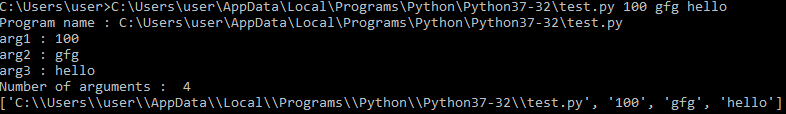Example 2 : This program demonstrates how command line arguments are passed into a function using sys.argv[index]. The command line arguments at index 1, 2 and 3 are stored into the variables arg1, arg2 and arg3. The variables arg1, arg2 and arg3 are passed to the function defined. However, the command line arguments can be passed directly without storing its value in local variables.

 `# importing the module ` `import` `sys ` ` `  `# function definition ` `def` `concat(s1, s2, s3): ` `    ``print``(s1 ``+` `" "` `+` `s2 ``+` `" "` `+` `s3) ` ` `  `# fetching the arguments ` `arg1 ``=` `sys.argv[``1``] ` `arg2 ``=` `sys.argv[``2``] ` `arg3 ``=` `sys.argv[``3``] ` ` `  `# calling the function ` `concat(arg1, arg2, arg3) `

Output :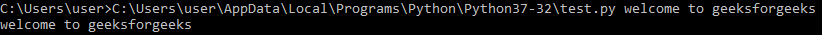Example 3 : This program demonstrates how command line arguments are passed into a function using sys.argv[index]. The command line arguments at index 1 and 2 are stored into the variables arg1 and arg2. The variables a and b are passed to the function defined. Command line arguments are accepted as strings, hence in order to perform numerical operations it should be converted into the desired numeric type first. For example, in this program the command line argument is converted into integer first and then stored into the variables. However, the command line arguments can be passed directly without storing its value in local variables.

 `# importing the module ` `import` `sys ` ` `  `# function definition ` `def` `add(a, b): ` `    ``print``(``"Result"``, a ``+` `b) ` ` `  `# fetching the arguments ` `arg1 ``=` `int``(sys.argv[``1``]) ` `arg2 ``=` `int``(sys.argv[``2``]) ` ` `  `# displaying the arguments ` `print``(arg1, arg2) ` ` `  `# calling the function ` `add(arg1, arg2) `

Output :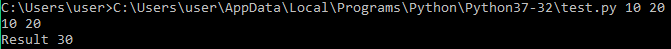Using argparse module
There is a certain problem with sys.argv method as it does not throw any specific error if the argument is not passed or argument of invalid type is passed. The argparse module gracefully handles the absence and presence of parameters. The following examples show the utility of argparse module:

 `# importing the module ` `import` `argparse ` ` `  `# creating an ArgumentParsert object ` `parser ``=` `argparse.ArgumentParser() ` ` `  `# fetching the arguments ` `parser.add_argument(``'number'``, ` `                    ``help` `=` `"Enter number to triple it."``) ` `args ``=` `parser.parse_args() ` ` `  `# performing some operation ` `print``(args.number ``*` `2``) `

Output :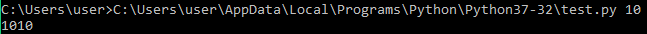Python by default accepts all command line arguments as string type hence the result is 55 ie. the string gets repeated twice. However, we can specify the data type we are expecting in the program itself so that whenever a command line argument is passed it is automatically converted into expected data type provided it is type compatible.

 `# importing the module ` `import` `argparse ` ` `  `# creating an ArgumentParsert object ` `parser ``=` `argparse.ArgumentParser() ` ` `  `# fetching the arguments ` `parser.add_argument(``'number'``, ` `                    ``help` `=` `"Enter number to double."``, ` `                    ``type` `=` `int``) ` `args ``=` `parser.parse_args() ` `print``(args.number ``*` `2``) `

Output :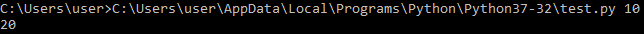We can look at the errors generated :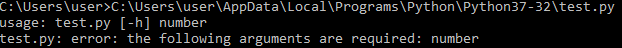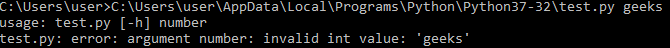This argparse throws specific error unlike the sys module. Any of the modules can be used as per convenience and requirement.

Python provides a getopt module that enables parsing command-line arguments.

Example :

 `import` `sys  ` `import` `getopt  ` `   `  `   `  `def` `full_name():  ` `    ``first_name ``=` `None` `    ``last_name ``=` `None` `   `  `    ``argv ``=` `sys.argv[``1``:]  ` `   `  `    ``try``:  ` `        ``opts, args ``=` `getopt.getopt(argv, ``"f:l:"``)  ` `       `  `    ``except``:  ` `        ``print``(``"Error"``)  ` `   `  `    ``for` `opt, arg ``in` `opts:  ` `        ``if` `opt ``in` `[``'-f'``]:  ` `            ``first_name ``=` `arg  ` `        ``elif` `opt ``in` `[``'-l'``]:  ` `            ``last_name ``=` `arg  ` `       `  `   `  `    ``print``( first_name ``+``" "` `+` `last_name)  ` `   `  `full_name() `

Output :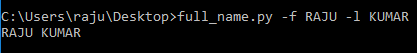My Personal Notes arrow_drop_upIf you like GeeksforGeeks and would like to contribute, you can also write an article using contribute.geeksforgeeks.org or mail your article to contribute@geeksforgeeks.org. See your article appearing on the GeeksforGeeks main page and help other Geeks.

Please Improve this article if you find anything incorrect by clicking on the "Improve Article" button below.

Article Tags :

Be the First to upvote.

Please write to us at contribute@geeksforgeeks.org to report any issue with the above content.